The OpenFOAM FoundationCatmullRomSpline Class Reference

An implementation of Catmull-Rom splines (sometimes known as Overhauser splines). More...

Inheritance diagram for CatmullRomSpline: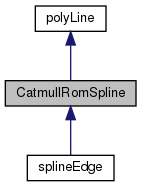[legend]
Collaboration diagram for CatmullRomSpline: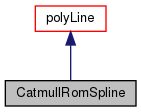[legend]

## Public Member Functions

CatmullRomSpline (const pointField &knots, const bool notImplementedClosed=false)
Construct from components. More...

CatmullRomSpline (const CatmullRomSpline &)=delete
Disallow default bitwise copy construction. More...

point position (const scalar lambda) const
Return the point position corresponding to the curve parameter. More...

point position (const label segment, const scalar lambda) const
Return the point position corresponding to the local parameter. More...

scalar length () const
Return the length of the curve. More...

void operator= (const CatmullRomSpline &)=delete
Disallow default bitwise assignment. More...Public Member Functions inherited from polyLine
polyLine (const pointField &, const bool notImplementedClosed=false)
Construct from components. More...

polyLine (const polyLine &)=delete
Disallow default bitwise copy construction. More...

const pointFieldpoints () const

label nSegments () const
Return the number of line segments. More...

point position (const scalar) const
Return the point position corresponding to the curve parameter. More...

point position (const label segment, const scalar) const
Return the point position corresponding to the local parameter. More...

scalar length () const
Return the length of the curve. More...

void operator= (const polyLine &)=delete
Disallow default bitwise assignment. More...Protected Member Functions inherited from polyLine
void calcParam ()
Precalculate the rational cumulative parameter value. More...

label localParameter (scalar &lambda) const
Return the line segment and the local parameter [0..1]. More...Protected Attributes inherited from polyLine
pointField points_
The control points or ends of each segments. More...

scalar lineLength_
The real line length. More...

scalarList param_
The rational (0-1) cumulative parameter value for each point. More...

## Detailed Description

An implementation of Catmull-Rom splines (sometimes known as Overhauser splines).

In this implementation, the end tangents are created automatically by reflection.

In matrix form, the local interpolation on the interval t=[0..1] is described as follows:

```P(t) = 1/2 * [ t^3 t^2 t 1 ] * [ -1  3 -3  1 ] * [ P-1 ]
[  2 -5  4 -1 ]   [ P0 ]
[ -1  0  1  0 ]   [ P1 ]
[  0  2  0  0 ]   [ P2 ]
```

Where P-1 and P2 represent the neighbouring points or the extrapolated end points. Simple reflection is used to automatically create the end points.

The spline is discretized based on the chord length of the individual segments. In rare cases (sections with very high curvatures), the resulting distribution may be sub-optimal.

A future implementation could also handle closed splines.

http://www.algorithmist.net/catmullrom.html provides a nice introduction
Source files

Definition at line 75 of file CatmullRomSpline.H.

## ◆ CatmullRomSpline() [1/2]

 CatmullRomSpline ( const pointField & knots, const bool notImplementedClosed = `false` )

Construct from components.

Definition at line 31 of file CatmullRomSpline.C.

## ◆ CatmullRomSpline() [2/2]

 CatmullRomSpline ( const CatmullRomSpline & )
delete

Disallow default bitwise copy construction.

## ◆ position() [1/2]

 Foam::point position ( const scalar lambda ) const

Return the point position corresponding to the curve parameter.

0 <= lambda <= 1

Definition at line 42 of file CatmullRomSpline.C.

Referenced by splineEdge::position().

Here is the call graph for this function: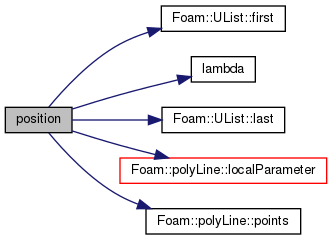Here is the caller graph for this function: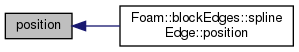## ◆ position() [2/2]

 Foam::point position ( const label segment, const scalar lambda ) const

Return the point position corresponding to the local parameter.

0 <= lambda <= 1 on the given segment

Definition at line 61 of file CatmullRomSpline.C.

Here is the call graph for this function: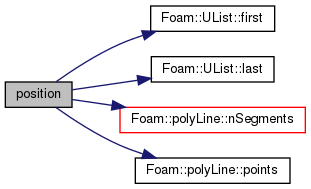## ◆ length()

 Foam::scalar length ( ) const

Return the length of the curve.

Definition at line 132 of file CatmullRomSpline.C.

References NotImplemented.

Referenced by splineEdge::length().

Here is the caller graph for this function: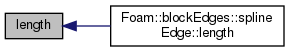## ◆ operator=()

 void operator= ( const CatmullRomSpline & )
delete

Disallow default bitwise assignment.

The documentation for this class was generated from the following files: